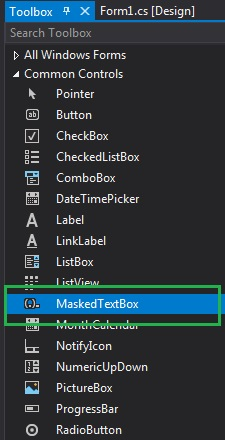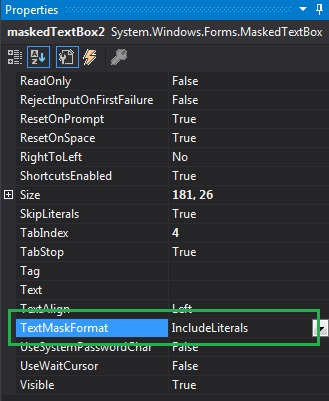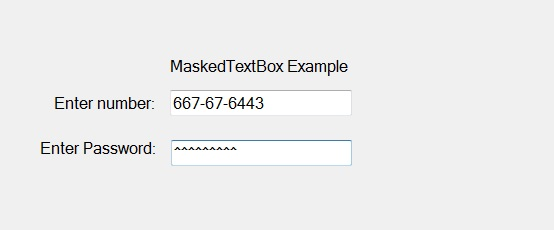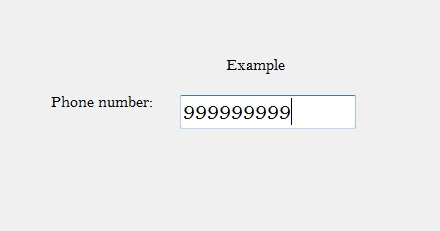Related Articles

# How to set the Text Mask Format of the MaskedTextBox in C#?

• Last Updated : 26 Jul, 2019

In C#, MaskedTextBox control gives a validation procedure for the user input on the form like date, phone numbers, etc. Or in other words, it is used to provide a mask which differentiates between proper and improper user input. In MaskedTextBox control, you set a value which indicates that the literals and the prompt characters are included in the formatted string present in the MaskedTextBox using TextMaskFormat Property provided by the MaskedTextBox control. This property has four values and these values are defined under MaskFormat enum and the values are:

• The ExcludePromptAndLiterals value returns the text input, which is provided by the user.
• The IncludeLiterals value returns the text input, which is provided by the user and the literal characters that are defined in the mask.
• The IncludePrompt value returns the text input, which is provided by the user and the instances of the prompt character.
• The IncludePromptAndLiterals value provides the text input, which is given by the user, the literal characters that are defined in the mask and the instances of the prompt character.

The default value of this property is IncludeLiterals. You can set this property in two different ways:

1. Design-Time: It is the easiest way to set the value of TextMaskFormat property of MaskedTextBox control as shown in the following steps:

• Step 1: Create a windows form as shown in the below image:

Visual Studio -> File -> New -> Project -> WindowsFormApp• Step 2: Next, drag and drop the MaskedTextBox control from the toolbox on the form. As shown in the below image:• Step 3: After drag and drop you will go to the properties of the MaskedTextBox and set the value of TextMaskFormat property of MaskedTextBox control as shown in the below image:Output:2. Run-Time: It is a little bit trickier than the above method. In this method, you can set the value of TextMaskFormat property of the MaskedTextBox control programmatically with the help of given syntax:

`public System.Windows.Forms.MaskFormat TextMaskFormat { get; set; }`

Here, MaskFormat holds the values of the TextMaskFormat property. If the value of this property does not belong to the MaskFormat enum, then it will throw an InvalidEnumArgumentException. The following steps show how to set the value of the TextMaskFormat property of the MaskedTextBox control dynamically:

```// Creating a MaskedTextBox
```
```// Setting the TextMaskFormat property
```
• Step 3: And last add this MaskedTextBox control to the form using the following statement:
```// Adding MaskedTextBox control on the form
```

Example:

 `using` `System;``using` `System.Collections.Generic;``using` `System.ComponentModel;``using` `System.Data;``using` `System.Drawing;``using` `System.Linq;``using` `System.Text;``using` `System.Threading.Tasks;``using` `System.Windows.Forms;`` ` `namespace` `WindowsFormsApp39 {`` ` `public` `partial` `class` `Form1 : Form {`` ` `    ``public` `Form1()``    ``{``        ``InitializeComponent();``    ``}`` ` `    ``private` `void` `Form1_Load(``object` `sender, EventArgs e)``    ``{`` ` `        ``// Creating and setting the ``        ``// properties of the Label``        ``Label l1 = ``new` `Label();``        ``l1.Location = ``new` `Point(413, 98);``        ``l1.Size = ``new` `Size(176, 20);``        ``l1.Text = ``" Example"``;``        ``l1.Font = ``new` `Font(``"Bell MT"``, 12);`` ` `        ``// Adding label on the form``        ``this``.Controls.Add(l1);`` ` `        ``// Creating and setting the``        ``// properties of Label``        ``Label l2 = ``new` `Label();``        ``l2.Location = ``new` `Point(242, 135);``        ``l2.Size = ``new` `Size(126, 20);``        ``l2.Text = ``"Phone number:"``;``        ``l2.Font = ``new` `Font(``"Bell MT"``, 12);`` ` `        ``// Adding label on the form``        ``this``.Controls.Add(l2);`` ` `        ``// Creating and setting the ``        ``// properties of MaskedTextBox``        ``MaskedTextBox m = ``new` `MaskedTextBox();``        ``m.Location = ``new` `Point(374, 137);``        ``m.Mask = ``"000000000"``;``        ``m.Size = ``new` `Size(176, 20);``        ``m.Name = ``"MyBox"``;``        ``m.BorderStyle = BorderStyle.Fixed3D;``        ``m.TextMaskFormat = MaskFormat.IncludeLiterals;``        ``m.Font = ``new` `Font(``"Bell MT"``, 18);`` ` `        ``// Adding MaskedTextBox``        ``// control on the form``        ``this``.Controls.Add(m);``    ``}``}``}`

Output:My Personal Notes arrow_drop_up